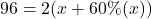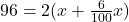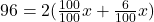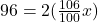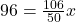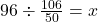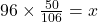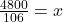## the shorter side of a rectangle is 60% of the longer side and the perimeter of the rectangle is 96 inches. find the side lengths

Question

the shorter side of a rectangle is 60% of the longer side and the perimeter of the rectangle is 96 inches. find the side lengths

in progress 0
6 months 2021-07-24T19:05:30+00:00 1 Answers 3 views 0

## Answers ( )

Length of the rectangle: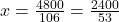Breadth of the rectangle: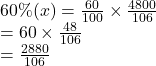Step-by-step explanation:

Longer side of the rectangle(length) = x

Shorter side of the rectangle(breadth) = (60%)x

Perimeter of the rectangle = 2(l+b) = 96 inches

Hence,